# Quiz 6: Booker Jones

Business

Calculation of "relevant" cost of manufacturing a challenger bike: Relevant cost here refers to "Variable cost" incurred on manufacturing a challenger bike which can be determined by summation of materials cost, labor cost, and overhead cost. Relevant cost is the cost considered for taking decision based on the cost to incurred, already incurred costs are not considered for decision making as these are termed as sunk costs and irrelevant. By extracting the information: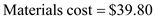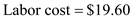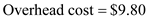Relevant cost of manufacturing a challenger bike can be calculated by adding materials cost, labor cost, and overhead cost incurred as follows: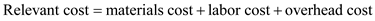Substitute:,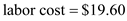, and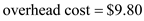Hence, the relevant cost of manufacturing a challenger bike comes out to be \$69.2.

Calculation of relevant cost (on per bicycle basis) of carrying the working capital investment involved in the Challenger deal: Relevant cost per bicycle of carrying the working capital investment involved the challenger deal can be calculated, as follows by using the information available from the case study: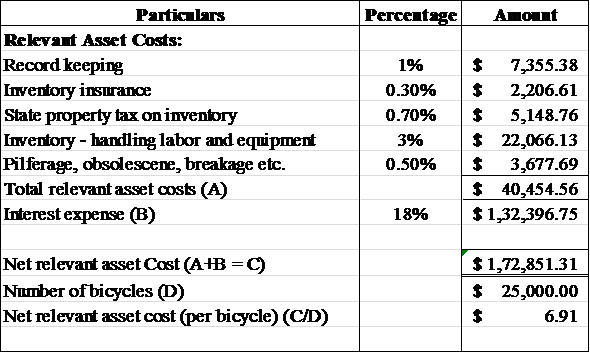Hence, from the above spreadsheet it can be said that the relevant cost per bicycle comes out to be \$6.91.

Yes, the challenger deal should be charged for the lost sale of bikes through regular distribution. Opportunity cost of the loss of sales of bikes should be considered for determining the feasibility of this decision. Calculation of contribution margin loss on 3000 units: Since the number of lost bikes is 3000 units, therefore contribution margin loss on 3000 units can be calculated as follows: Step 1 Calculation of the material cost: By extracting information: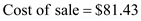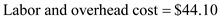Material cost can be calculated as follows: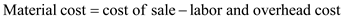Substitute: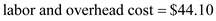Hence, balance material cost is \$37.33. Step 2 Calculation of variable cost of regular bike: Variable cost of regular bike can be calculated by using the following formula: Substitute: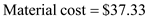,and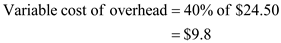Hence, the variable cost of a regular bike comes out to be \$66.73. Step 3 Calculation of contribution margin per unit: Contribution margin per unit can be calculated by using the following formula: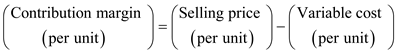Selling price per unit in case of regular bikes is \$110.05. Substitute: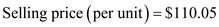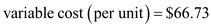Hence, contribution margin per unit comes out to be \$43.32. Step 4 Calculation of contribution margin loss on 3000 units and total opportunity cost: Contribution margin loss on 3000 units can be calculated by using the following formula: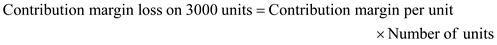Substitute: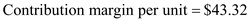and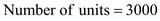Hence, contribution margin loss on 3000 units comes out to be \$129,960. Therefore, total opportunity cost can be determined by adding one time added cost of \$5000 to the contribution margin loss of \$129,960 and hence it comes out to be \$134,960.